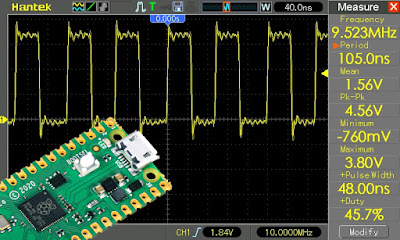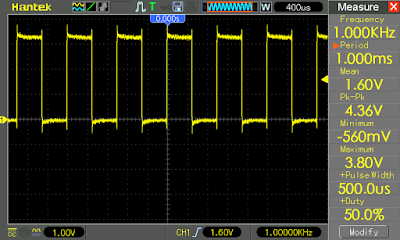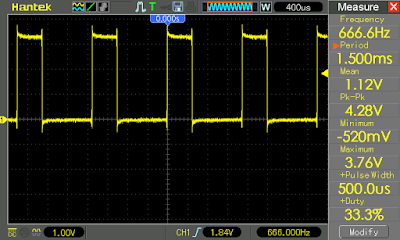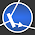### Generate square wave signal with Raspberry Pi Pico PIO

The MCU of Raspberry Pi Pico contains a special I/O unit which can be programmed to emulate custom protocols

I recently bought a couple of Raspberry Pi Pico microcontroller boards. Although I read about the PIO peripheral, I didn't pay too much attention to it. However, it seems to be an interesting peripheral that I haven't seen before on other microcontrollers. It is supposed to be a versatile I/O interface which will allow you to implement custom serial or parallel protocols in a better way than bitbanging a GPIO pin.

Actually, the PIO is made of two blocks, each containing four state machines. These are individual processing units optimized for I/O, with "a focus on determinism, precise timing, and close integration with fixed-function hardware" as the datasheet claims. Sounds good, doesn't it? This is until you get to program these "machines" in... assembly language. I'm totally new to this, so in this post I will generate a square wave signal using the PIO.It is very easy to toggle a pin in MicroPython or C/C++. But you never get enough control over the frequency and duty cycle when you loop toggle a pin. There are delays to reduce frequency, but they are blocking. One may also generate square wave signals and PWM signals by using a timer. Raspberry Pi Pico has a dedicated PWM peripheral and also timers, but for the purpose of learning something, I will do it with PIO. Especially because I didn't quite find a detailed documentation for the state machines. And, as the datasheet says: "the PIO is much more flexible when programmed directly, supporting a wide variety of interfaces which may not have been foreseen by its designers".

from machine import Pin
from rp2 import PIO, StateMachine, asm_pio

@asm_pio(set_init=PIO.OUT_LOW)
def square():
wrap_target()
set(pins, 1)
set(pins, 0)
wrap()

sm = rp2.StateMachine(0, square, freq=2000, set_base=Pin(2))

sm.active(1)

We import the required Python classes and define a function which will "operate" the state machine. That is def square():. Note that it is preceded by a decorator which informs the Python interpreter that what comes next contains assembly. There is more to @asm_pio, but for now all we do is set the initial state of the I/O as output, low. Program configuration is set in this decorator.

Let's skip the assembly for now and move to state machine init. This is the line:

sm = rp2.StateMachine(0, square, freq=2000, set_base=Pin(2))

I selected state machine 0 (first argument), I want it to execute the code from square function while running at a clock frequency of 2 kHz. The last argument specifies that the I/O manipulation applies to GP2 (pin 4 on the board). Remember that pins and frequency are set here. I could have used GP25 for the built-in LED, but I found it easier to hook pin 4 to the oscilloscope. Okay, let's look at the assembly code:

def square():
wrap_target()
set(pins, 1)
set(pins, 0)
wrap()

Basically, I set pin to 1 then to 0. These two instructions are included in a wrap command. Each of the set instructions takes one program cycle to execute. wrap resets program counter and starts over again. So, if the state machine is clocked at 2 kHz, I should get a 1 kHz square wave? Exactly!1 kHz square wave produced by state machine

But what are the limits of the state machine clock frequency? For these two instructions, it wasn't able to go to less than 2 kHz (going below still produced a waveform of 1 kHz). Let's reduce the output frequency by half. There are two ways to do this:

def square():
wrap_target()
set(pins, 1) 
set(pins, 0) 
wrap()

The  tells the state machine to do nothing for the next cycle. I could have used even a higher number of cycles. Or, if you prefer, you may add nop() instructions. Let's test the following code:

def square():
wrap_target()
set(pins, 1)
nop()
set(pins, 0)
nop() 
wrap()

Can you guess the frequency and duty cycle? It's easy: set to 1 and nop take two cycles. set to 0, nop and  take three cycles. So, two cycles high and three cycless low gives a duty cycle of 40%/60%. And each instruction is executed by the state machine with a frequency of 2 kHz. Five instructions mean the square wave will perform a full cycle in 2 kHz divided by 5, or 400 Hz. The oscilloscope is here for confirmation ;)40% duty cycle 400 Hz wave generated by state machine

By now, I know the lowest state machine frequency is 2 kHz. But how high can we go? I was able to get a relatively good-looking square wave of 10 MHz with the state machine set to 20 MHz. Look at the top image of this post (with a 10 MHz signal) and note that it is already a bit "wobbled". You can go higher, but strange waveforms will be generated (as a side note, some signal distortions may occur due to my oscilloscope)."Square wave" output when state machine frequency set to 80 MHz

There is one more thing I have to mention. Let's replace wrap with a jmp instruction.

def square():
label("start")
set(pins, 1)
set(pins, 0)
jmp("start")

Did you expect a 50% duty signal?Wave output with jump instruction

Well, no. The jump instruction takes one clock cycle (after all it is a conditional instruction, although I did not make use of a condition here). So, for one cycle, the output is set to high. The next cycle, it is set to low and remains so while the jump is executed. Note the frequency divided by three (state machine configured for 2 kHz). Do you want the 50% duty cycle back? Here is a way to get it:

def square():
label("start")
set(pins, 1) 
set(pins, 0)
jmp("start")

You have to double state machine frequency to get the initial square wave frequency. I'll end this post here. The PIO is a unique, versatile peripheral of Raspberry Pi Pico MCU. Using assembly to program it is not quite straightforward, yet there are definitely advantages over bitbanging. In future posts I will take control of multiple pins assigned to the same state machine.

1.hello,

this code:

from machine import Pin
from rp2 import PIO, StateMachine, asm_pio

@asm_pio(set_init=PIO.OUT_LOW)
def square():
wrap_target()
set(pins, 1)
set(pins, 0)
wrap()

sm = rp2.StateMachine(0, square, freq=2000, set_base=Pin(2))

sm.active(1)

NOT working ?? Where I do error?
Thonny IDE write:

Traceback (most recent call last):
File "", line 5, in
File "rp2.py", line 228, in asm_pio
File "rp2.py", line 36, in __init__
ImportError: no module named 'array'

1.2.Hello, nice and working code Is It a way to compile It in Circuit Python ?
Thank you !

1.2.Yes, CircuitPython has support for state machine programming. However, the code must be modified to be able to compile it.

3.Perfect! I needed a nice signal to test my old CRO, and figured the Pi Pico PIO would be the easiest and cleanest way.
Couldn't have been easier than this. Thanks for the tutorial.

4.Thank you for the great example! Can 4 IO from the PIO state machine be used as a quadrature encoder input? That would be a fantastic example.